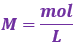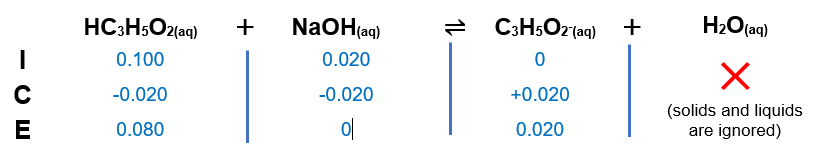# Problem: Calculate the pH after 0.020 mole of NaOH is added to 1.00 L of each of the following solutions:a. 0.100 M propanoic acid (HC3H5O2, Ka = 1.3 x 10-5)b. 0.100 M sodium propanoate (NaC3H5O2)

###### FREE Expert Solution

We are asked to calculate the pH after 0.020 mole of NaOH is added to 1.00 L of each of the following solutions

a. 0.100 M propanoic acid (HC3H5O2, Ka = 1.3 x 10-5)

Step 1. Calculate the initial amounts of HC3H5O2 in moles before the reaction happens.

molarity (volume) → moles

Recall:*convert volumes from mL to L → 1 mL = 10-3 L

1.00 L of 0.100 M HC3H5O2

mol HC3H5O2 = 0.100 mol  HC3H5O2

Step 2. Write the chemical equation for the reaction between HC3H5O2 and NaOH.

Reaction:

HC3H5O2(aq)    +   NaOH(aq)               C3H5O2(aq)       +       H2O(aq)
(weak acid)          (base)                    (conjugate base)     (conjugate acid)

Step 3. Construct an ICF Chart.Step 4. Calculate pH.

Since we have a buffer, we can calculate the pH of the solution using the Henderson-Hasselbalch equation:

pH = 4.28

The resulting solution after the titration has a pH of 4.28.

92% (228 ratings)###### Problem Details

Calculate the pH after 0.020 mole of NaOH is added to 1.00 L of each of the following solutions:

a. 0.100 M propanoic acid (HC3H5O2, Ka = 1.3 x 10-5)
b. 0.100 M sodium propanoate (NaC3H5O2)

Frequently Asked Questions

What scientific concept do you need to know in order to solve this problem?

Our tutors have indicated that to solve this problem you will need to apply the Buffer concept. You can view video lessons to learn Buffer. Or if you need more Buffer practice, you can also practice Buffer practice problems.

What professor is this problem relevant for?

Based on our data, we think this problem is relevant for Professor Taylor's class at CLEMSON.

What textbook is this problem found in?

Our data indicates that this problem or a close variation was asked in Chemistry: An Atoms First Approach - Zumdahl Atoms 1st 2nd Edition. You can also practice Chemistry: An Atoms First Approach - Zumdahl Atoms 1st 2nd Edition practice problems.